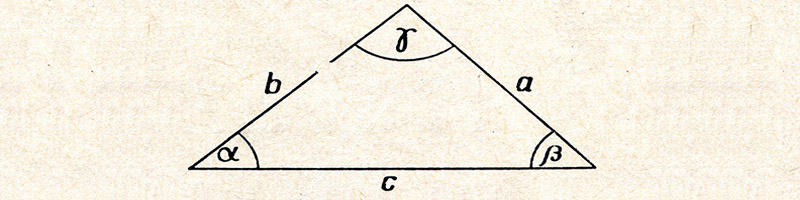# Geometry

Satellite based GPS systems, physicists and architects all use geometry.  Most students begin to understand how geometry intersects with life through their understanding of planes, lines, angles and points. As they learn how to apply theorems and deduct proofs they build an essential foundation for spatial reasoning and mathematical understanding.

#### General Overview of Geometry Content

(Content varies across curricula.)

• Points, Lines, Angles, Planes
• Reasoning & Proof: Inductive, Deductive & Conditional Statements
• Parallel & Perpendicular Lines: Angles, Transversals, Slope

• Congruent Triangles: SSS, SAS, ASA, AAS
• Relationships with Triangles, Perpendicular Bisectors
• Similarity: Geometric Mean, Polygons, Theorems

• Right Triangles & Trigonometry
• Quadrilaterals: Parallelograms, Rhombuses, Rectangles and Squares
• Properties of Transformations: Vectors, Matrices, Reflections, Rotations, Dilations, Transformations

• Properties of Circles: Tangents, Arc Measures, Properties of Chords
• Measuring Length & Area: Circumference, Rhombuses, Kites
• Surface Area & Volume of Solids: Prisms, Cylinders, Pyramids, Spheres, Cones